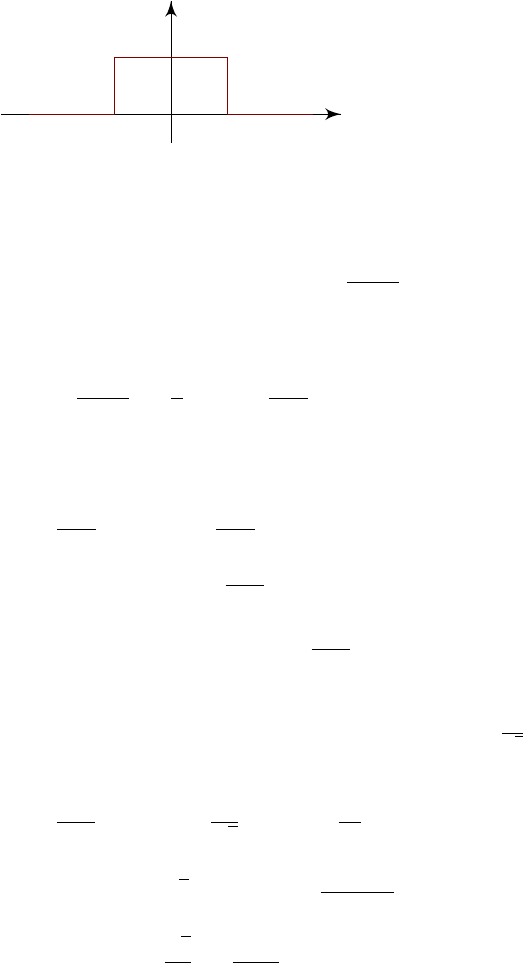6Fourier transforms

IB Methods6.4 A word of warning
Suppose f(x) is defined by
f(x) =
(
1 |x| < 1
0 |x| 1.
x
y
This function looks rather innocent. Sure it has discontinuities at
±
1, but these
are not too absurd, and we are just integrating. This is certainly absolutely
integrable. We can easily compute the Fourier transform as
˜
f(k) =
Z
−∞
e
ikx
f(x) dx =
Z
1
1
e
ikx
dx =
2 sin k
k
.
Is our original function equal to the integral F
1
[
˜
f(l)]? We have
F
1
2 sin k
k
=
1
π
Z
−∞
e
ikx
sin k
k
dk.
This is hard to do. So let’s first see if this function is absolutely integrable. We
have
Z
−∞
e
ikx
sin k
k
dx =
Z
−∞
sin k
k
dk
= 2
Z
0
sin k
k
dk
2
N
X
n=0
Z
(n+3/4)π
(n+1/4)π
sin k
k
dk.
The idea here is instead of looking at the integral over the whole real line, we
just pick out segments of it. In these small segments, we know that
|sin k|
1
2
.
So we can bound this by
Z
−∞
e
ikx
sin k
k
dx 2
N
X
n=0
1
2
Z
(n+3/4)π
(n+1/4)π
dk
k
2
N
X
n=0
Z
(n+3/4)π
(n+1/4)π
dk
(n + 1)π
=
2
2
N
X
n=0
1
n + 1
,
and this diverges as
N
. So we don’t have absolute integrability. So we
have to be careful when we are doing these things. Well, in theory. We actually
won’t. We’ll just ignore this issue.